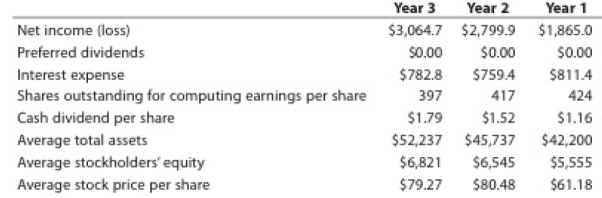Chapter 17, Problem 17.5CP

Chapter
Section
Textbook Problem

Profitability analysisDeere & Company manufactures and distributes farm and construction machinery that it sells around the world. In addition to its manufacturing operations, Deere’s credit division loans money to customers to finance the purchase of their farm and construction equipment.The following information is available for three recent years (in millions except per-share amounts):1. Calculate the following ratios for each year, rounding ratios and percentages to one decimal place, except for per-share amounts: a. Return on total assets b. Return on stockholders’ equity c. Earnings per share d. Dividend yield e. Price-earnings ratio 2. Based on these data, evaluate Deere’s profitability.

To determine

Financial Ratios: Financial ratios are the metrics used to evaluate the liquidity, capabilities, profitability, and overall performance of a company.

Given info: Items of financial statement

To determine: The following ratios for three years:

1. (a) Return on total assets
2. (b) Return on stockholders’ equity
3. (c) Earnings per share
4. (d) Dividend yield
5. (e) Price – earnings ratio
Explanation

Return on assets determines the particular company’s overall earning power. It is determined by dividing sum of net income and interest expense and average total assets.

Formula:

Rate of return&#

2.

To determine

To evaluate: D’s profitability

Still sussing out bartleby?

Check out a sample textbook solution.

See a sample solution

The Solution to Your Study Problems

Bartleby provides explanations to thousands of textbook problems written by our experts, many with advanced degrees!

Get Started

Find more solutions based on key concepts